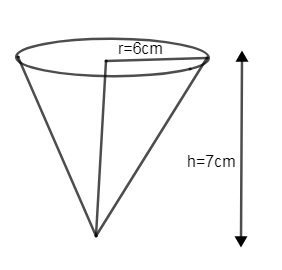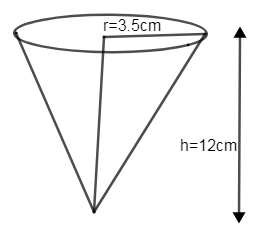Courses
Courses for Kids
Free study material
Free LIVE classes
MoreLIVE
Join Vedantu’s FREE Mastercalss

# Find the volume of the right circular cone with(i) radius 6cm, height 7cm.(ii) radius 3.5cm, height 12cm.Verified
360.6k+ views
Hint: Draw the figure of a right circular cone for both the cases with a given radius and height. Substitute the value in the formula for volume of the cone. Cancel out like terms and find the volume of the cone for both the cases.

Let us assume the value. $\pi =\dfrac{22}{7}\approx 3.14$Consider the given figure as a right circular cone with height of 7cm and radius, r of 6cm.
We know the volume of a cone $=\dfrac{1}{3}\pi {{r}^{2}}h$$c{{m}^{3}}$.
We know the value of r and h.
r = 6cm, h = 7cm.
$\therefore$ Volume of the cone, $V=\dfrac{1}{3}\pi {{r}^{2}}h=\dfrac{1}{3}\pi \times {{\left( 6 \right)}^{2}}\times \left( 7 \right)$
$=\dfrac{1}{3}\times \dfrac{22}{7}\times 36\times 7$.
Cancel out the like terms,
$=22\times 12=264 c{{m}^{3}}$Consider the given figure as a right circular cone with height 12 cm and radius, r of 3.5cm.
We know the volume of cone $=\dfrac{1}{3}\pi {{r}^{2}}h$$c{{m}^{3}}$.
We know the value of r and h.
r = 3.5cm, h = 12cm.
$\therefore$ Volume of the cone, $V=\dfrac{1}{3}\pi {{r}^{2}}h=\dfrac{1}{3}\pi \times {{\left( 3.5 \right)}^{2}}\times \left( 12 \right)$
$=\dfrac{1}{3}\times \dfrac{22}{7}\times 12.25\times 12$
Cancel out the like terms.
$=\dfrac{22}{7}\times 12.25\times 4=154 c{{m}^{3}}$
$\therefore$ We got the volume of the right circular cone with radius 6 cm and height 7 cm as $264 c{{m}^{3}}$.
The volume of the right circular cone with radius 3.5cm and height 12 cm as $154 c{{m}^{3}}$.

Note: The capacity of a cone is $\dfrac{1}{3}$rd the capacity of a cylinder, which means that is we take $\dfrac{1}{3}$rd of volume of a cylinder, we get the formula for the volume of a cone, having the same radius and height.
$\therefore$Volume, $V=\dfrac{1}{3}\times$ area of the circular base $\times$ height of the cone
The formula for area of the circular base $=\pi {{r}^{2}}$ and height h,
$V=\dfrac{1}{3}\pi {{r}^{2}}h$
Hence, V is the volume, r is the radius and h is the height.
Last updated date: 21st Sep 2023
Total views: 360.6k
Views today: 5.60k